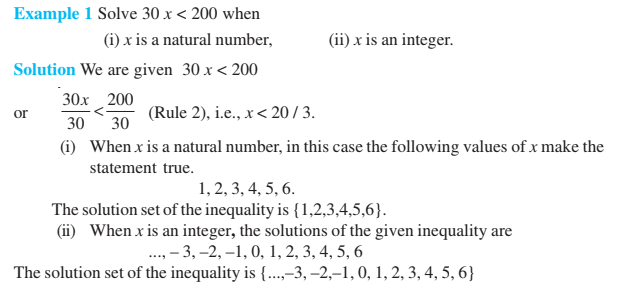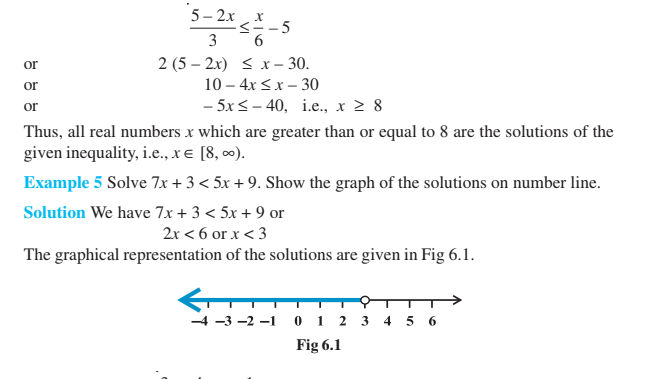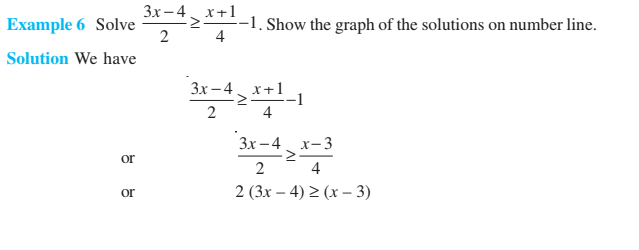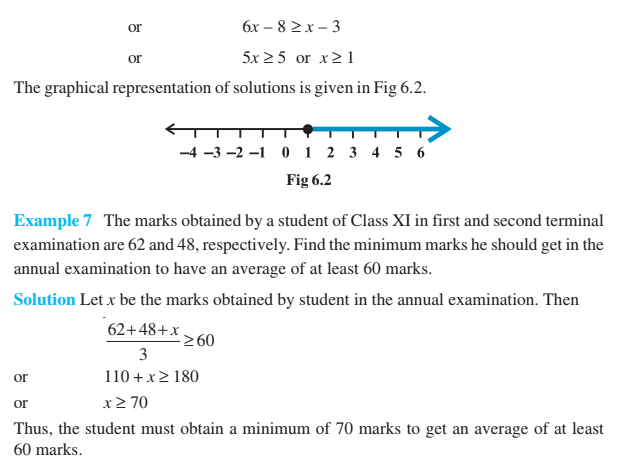# Linear Inequalities Class 11

Linear Inequalities Class 11 Concept: Two algebraic expressions or real numbers related by the symbol ≤, ≥, < and > form an inequality. For example: px + qy > 0, 9a – 21b < 0, etc. From equality Equal numbers can be subtracted or added from both the sides of an equation. Also, both sides of an inequality can be divided or multiplied by the same number (non-zero). The notes are provided here for students to have a reference source while preparing for the exam. Solve the extra questions based on the methods provided by us here to understand the concept thoroughly.

1. Solve 4x + 3 < 6x +7

Solution: Given, 4x + 3 < 6x +7

Therefore, 3 – 7 < 6x – 4x or, 2x > – 4, i.e. x > -2

Hence, the solution of the given inequality is (–2, ∞).

2. Solve $\frac{5-2x}{3}\leq \frac{x}{6}-5$

Solution: Given $\frac{5-2x}{3}\leq \frac{x}{6}-5$

Therefore, 10-4x ≤ x-30

Or, -5x ≤ -40 or x ≥ 8

Therefore, all real numbers equal to or greater than 8 are the solutions of the given inequality i.e. x ∈ [8, ∞]

## Linear Inequalities class 11 Examples

The examples based on the concept linear inequalities are solved here for better understanding. Students can follow each and every step and try to solve the problems. Basically the symbols such as ≤ (less than and equal to), ≥ (greater than and equal to), < (less than) and > (greater than) are used in between the equations. We have to solve all such equations, maintaining the meaning of the statement. Now, let us see some examples here.To learn all concepts in Math in a more engaging way, register at BYJU’S. Also, watch interesting videos on various maths topics by downloading BYJU’S– The Learning App from Google Play Store or the app store.